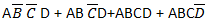# The output of the circuit will be

1.

2.

3.

4.

4Explanation :
No Explanation available for this question

# The type of gate shown in the given figure is

1.  NOR

2.  OR

3.  NAND

4.  EX-OR

4

EX-OR

Explanation :
No Explanation available for this question

# A second-order system has a transfer function given by G(s)=25/s2+8s+25. If the system, initially at rest, is subjected to a unit step input at t=0, the second peak in the response will occur at

1.  πsec

2.   π/3sec

3.  2π/3sec

4.   π/2sec

4

πsec

Explanation :
No Explanation available for this question

# A unity –feedback control system has the open-loop transfer function G(s)=4(1+2s)/s2(s+2). If the input to the system is a unit ramp, the steady-state error will be

1.   0

2.  0.5

3.   2

4.  Infinity

4

0

Explanation :
No Explanation available for this question

# The open-loop transfer function of a dc motor is given as( ω(s)/Va(s))=10/1+10s. When connected in feedback as shown below, the approximate value of Ka that will reduce the time constant of the closed loop system by one hundred times as compared to that of the open-loop system is

1.   1

2.   5

3.  10

4.  100

4

10

Explanation :
No Explanation available for this question

# In the logic circuit of given figure, redundant gate is

1.  1

2.  2

3.  3

4.  4

4

2

Explanation :
No Explanation available for this question

# Which one of the following statements is NOT TRUE for a continuous time causal and stable LTI system

1.   All the poles of the system must lie on the left side of the jω axis

2.  Zeros of the system can lie anywhere in the s-plane

3.  All the poles must lie within

4.  All the roots of the characteristic equation must be located on the left side of the jω axis

4

All the poles must lie within

Explanation :
No Explanation available for this question

# A polynomial f(x)=a4x4 + a3x3+a2x2+a1x-a0 with all coefficients positive has

1.   No real roots

2.   No negative real root

3.   Odd number of real roots

4.  At least one positive and one negative root

4

At least one positive and one negative root

Explanation :
No Explanation available for this question

# An electromechanical closed-loop control system has the following characteristic equation s3+6Ks2+(K+2)s+8=0 where K is the forward gain of the system. The condition for closed loop stability is

1.   K-0.528

2.  K-2

3.   K=0

4.  K=-2.258

4

K-2

Explanation :
No Explanation available for this question

# The feedback control system in the figure is stable

1.   For all k≥0

2.  Only if k≥1

3.  Only if 0≤k

4.   Only if 0≤k≤2

4